# Brouwer lattice

(diff) ← Older revision | Latest revision (diff) | Newer revision → (diff)
A distributive lattice in which for each pair of elementsthere exists an element, called the pseudo-difference (frequently denoted by), which is the smallest elementpossessing the property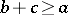. An equivalent description of a Brouwer lattice is as a variety of universal algebras (cf. Universal algebra) with three binary operations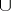,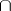and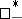, which satisfies certain axioms. The term "Brouwer algebra" was introduced in recognition of the connection between Brouwer lattices and Brouwer's intuitionistic logic. Instead of Brouwer lattices the so-called pseudo-Boolean algebras are often employed, the theory of which is dual to that of Brouwer lattices. Any Brouwer lattice can be converted to a pseudo-Boolean algebra by the introduction of a new order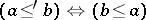, and of new unions and intersections according to the formulas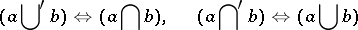and the operation of relative pseudo-complementation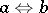which corresponds to the pseudo-difference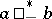. Conversely, any pseudo-Boolean algebra can be regarded as a Brouwer lattice. The term "Brouwer lattice" is sometimes used to denote a pseudo-Boolean algebra (see, for instance, ).